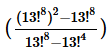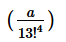# Test: The Powerful

## 15 Questions MCQ Test Quantitative Aptitude for GMAT | Test: The Powerful

Description
Attempt Test: The Powerful | 15 questions in 30 minutes | Mock test for GMAT preparation | Free important questions MCQ to study Quantitative Aptitude for GMAT for GMAT Exam | Download free PDF with solutions
QUESTION: 1

Solution:
QUESTION: 2

Solution:
QUESTION: 3

### How many terminating zeroes does 200! have?

Solution:
QUESTION: 4

If x is a positive integer, what is the units digit of x2?

1) The units digit of x4 is 1
2) The units digit of x is 3

Solution:
QUESTION: 5

If p is a positive integer, and x = m1/3, y = n1/2, and z = 2p, then which one of x, y, and z is the greatest?

1)  4p3 = 5m
2)  5n = 3p

Solution:
QUESTION: 6

Is ax equal to 4?

1)  ax + 1 = 4
2)  (a + 1)x = 4

Solution:
QUESTION: 7

How many different positive integers exist between 106 and 107, the sum of whose digits is equal to 2?

Solution:

Method 1 to solve this GMAT Number Properties Question: Find the number of such integers existing for a lower power of 10 and extrapolate the results.
Between 10 and 100, that is 101 and 102, we have 2 numbers, 11 and 20.
Between 100 and 1000, that is 102 and 103, we have 3 numbers, 101, 110 and 200.
Therefore, between 106 and 107, one will have 7 integers whose sum will be equal to 2.

Alternative approach
All numbers between 106 and 107 will be 7 digit numbers. There are two possibilities if the sum of the digits has to be '2'.
Possibility 1: Two of the 7 digits are 1s and the remaining 5 are 0s. The left most digit has to be one of the 1s. That leaves us with 6 places where the second 1 can appear.
So, a total of six 7-digit numbers comprising two 1s exist, sum of whose digits is '2'.
Possibility 2: One digit is 2 and the remaining are 0s. The only possibility is 2000000. Total count is the sum of the counts from these two possibilities = 6 + 1 = 7

QUESTION: 8

If= a, what is the units digits of?

Solution:

Take common (13!^8) from numerator
take common (13!^4) from denominator

now 13! has last digit 0
so 0-1 =-1=10+(-1)=9

Hence Option D is correct

QUESTION: 9

Is x > 1010?

1) x > 234
2) x = 235

Solution:
QUESTION: 10

If x = 232 * 254 * 276 * 298 and x is a multiple of 26n where n is a non-negative integer, then what is the value of n26 – 26n?

Solution:
QUESTION: 11

If x is a positive integer, what is the remainder when 712x + 3 + 3 is divided by 5?

Solution:
QUESTION: 12

In which of the following choices must p be greater than q?

Solution:
QUESTION: 13

What is the greatest prime factor of 417 – 228?

Solution:
QUESTION: 14

How many keystrokes are needed to type numbers from 1 to 1000?

Solution:

While typing numbers from 1 to 1000, there are 9 single digit numbers: from 1 to 9.
Each of these numbers requires one keystroke.
That is 9 key strokes.
There are 90 two-digit numbers: from 10 to 99.
Each of these numbers requires 2 keystrokes.
Therefore, 180 keystrokes to type the 2-digit numbers.
There are 900 three-digit numbers: from 100 to 999.
Each of these numbers requires 3 keystrokes.
Therefore, 2700 keystrokes to type the 3-digit numbers.
1000 is a four-digit number which requires 4 keystrokes.
Totally, therefore, one requires 9 + 180 + 2700 + 4 = 2893 keystrokes.

QUESTION: 15

Positive integers a, b, c, m, n, and p are defined as follows: m = 2a3b, n = 2c, and p = 2m/n, is p odd?

1) a < b
2) a < c

Solution:Use Code STAYHOME200 and get INR 200 additional OFF Use Coupon Code